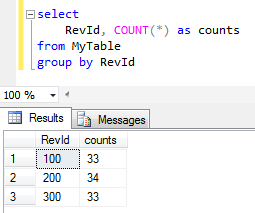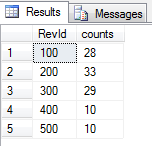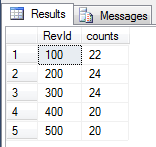SQL Server and T-SQL Development Tutorials Development resources, articles, tutorials, code samples and tools and downloads for ASP.Net, SQL Server, R Script, Windows, Windows Phone, AWS, SAP HANA and ABAP, like SAP UI5, Screen Personas, etc.
 Home Articles News IT Jobs Tools Sample Chapters Trainers Blogs Forums Photos Files

SQL Server 2019 Installation

# Update Table Data for Uniform Distribution in SQL

On SQL Server database table, if SQL developers want to create equal number of rows or uniform distribution among data categories database programmers can use SQL script given in this tutorial.

The original SQL table data is having a distribution on selected column as follows``` ;with summary as (  SELECT distinct   COUNT(*) Over (Partition By 1) Cnt,   RevId,   COUNT(RevId) Over (Partition By RevId) RevCnt  FROM MyTable ), todo as (  select   Cnt,   Cnt / (count(*) over (partition by 1)) Average,   RevId, RevCnt  from summary ), joint as ( select  id,  MyTable.RevId,  orderno = ROW_NUMBER() over (partition by MyTable.RevId order by id),  Cnt,  Average,  RevCnt,  RevCnt - Average as forupdate from MyTable inner join todo on MyTable.RevId = todo.RevId ), upd as (  select * from joint  where RevCnt < Average and orderno <= (forupdate * -1)  union all  select * from joint  where RevCnt > Average and orderno <= forupdate ), final as ( select  id, RevId,  case when forupdate > 0 then 1 else -1 end as overAvg,  rn = ROW_NUMBER() over (partition by (case when forupdate > 0 then 1 else -1 end) order by id) from upd ) update MyTable set  RevId = ISNULL(n.RevId,p.RevId) from MyTable t inner join final p on p.id = t.id left join final n on p.rn = n.rn and n.overAvg = -1 where p.overAvg = 1 ```

After above SQL update statement is executed, table data is now having a uniform distribution on selected columnUnfortunately, I realized that above update is not sufficient in some cases.
Assume that our values are distributed as seen in below numbers in our sample database table.

As you will realize, I had a total number of 100 rows at first sample.
Then for following case, I had introduced two new groups of rows in low numbers.
5 new rows for category 400 and 5 rows for category group 500After we execute above code, the updated data will have below count values.
Since the count difference between minimum group (with 5 rows) and target distribution count (100+5+5)/5 = 22 is bigger than minimum group count (5), the distribution is not uniform even after first correction.An other attemp to make numbers equal among different categories or groups by running the same script once more will improve the resultIf not satistied with the result, let's try again. Execute the exact SQL script again. And then check the final situation on value distribution on table data.Yes now it is all uniformly distributed :)

Related SQL Resources

SQL Server Articles

SQL Server 2012

SQL Server Tools

SQL Blog

MS SQL Server Forums

## SQL Split String Related SQL Server Tutorials

Split String using XML - How to Convert or Split a Delimited String Values to Rows using T-SQL XML Commands
Split String Into Fixed Length Pieces in SQL
Recursive Function Sample - MS SQL Server Recursive T-SQL Split Function
TSQL Character Split Function in SQL Server
Case Sensitive SQL Split Function
SQL Server 2016 Split String Function STRING_SPLIT
SQL Server String Split T-SQL CLR Function Sample
SQL Server CLR Split String Function for 2-Dimensional Array
T-SQL Split User Defined Function